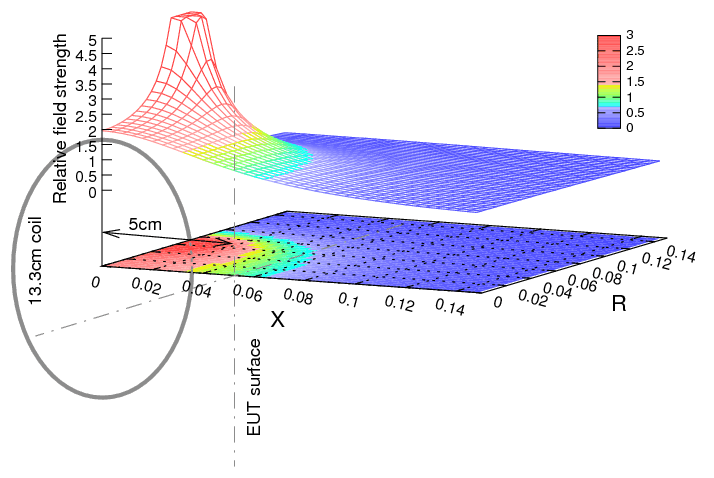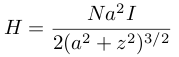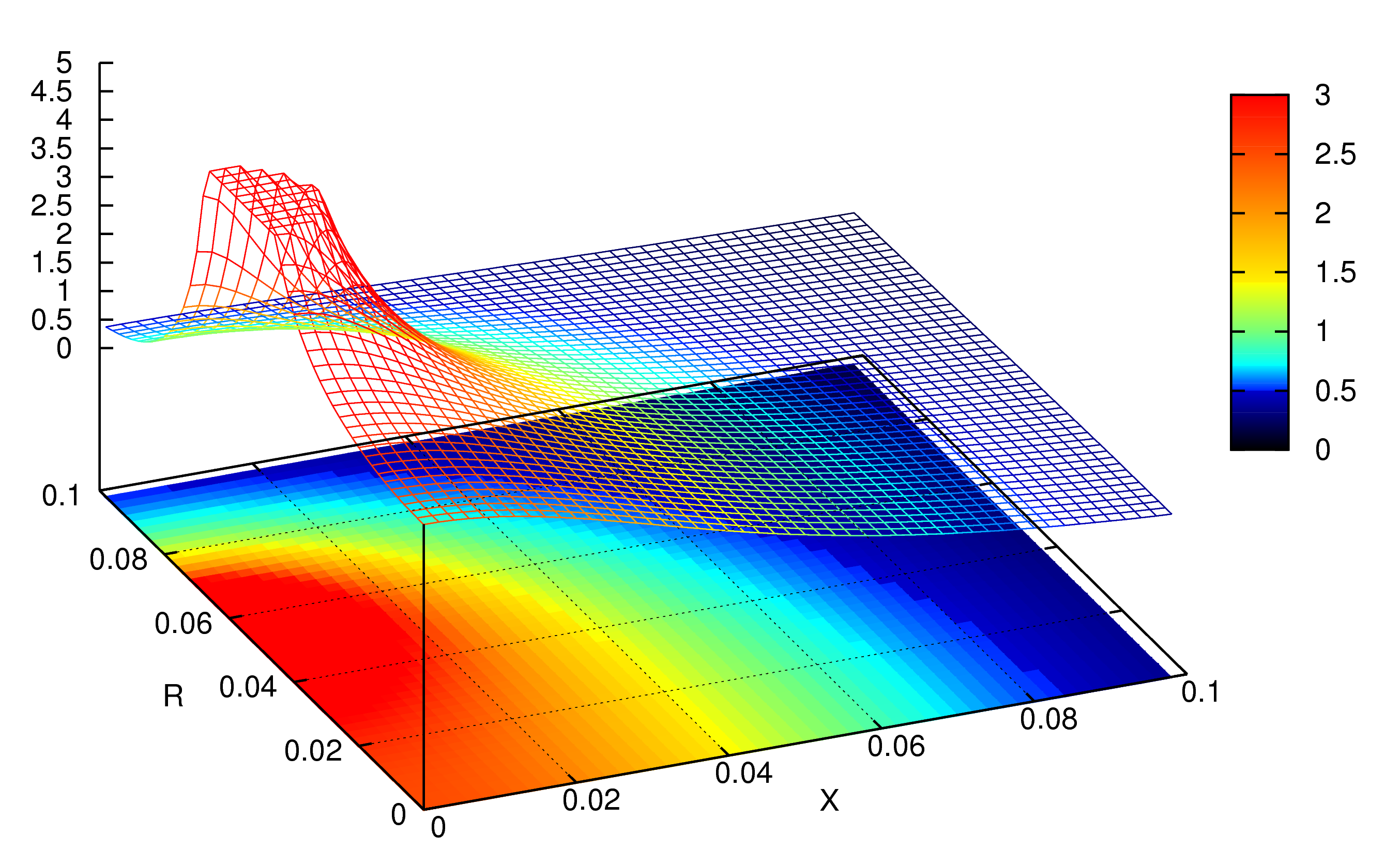[ Home > Magnetic field from a 13.3cm circular radiating coil ]

# Magnetic field from a 13.3cm and 12cm circular radiating coil

## Magnetic field from a 13.3cm circular radiating coil

Note: this graph shows calculated magnetic field strength distribution, relative to the value at 5cm on the axis from center of the coil. The coil diameter of 13.3cm and the reference point of 5cm are those used for EN 55103-2 low frequency magnetic field immunity tests.Magnetic field strength H (A/m) on the center axis of the coil can be calculated from:Here, a is the coil radius (m), N is the number of turns, and z is the distance from center of the coil.

For a = 0.133 / 2 + 0.00125 × 2, N = 36 and z = 0.05, it calculates H ≅ 138.5 I. For a = 0.12 / 2, N = 20 and z = 0.05, it calculates H ≅ 76.8 I.

## Magnetic field from a 12cm circular radiating coilThe coil is centered at X = 0m and R = 0m, and the coil winding is at X = 0m and R = 0.06m. DUT will be placed at X = 0.05m.

## Reference

• Off-axis field due to a current loop, Eric Dennison, 2004
• EN 55103-2, Electromagnetic compatibility - Product family standard for audio, video, audio-visual and entertainment lighting control apparatus for professional use - Immunity,
• ISO 11452-8, Road vehicles – Component test methods for electrical disturbances by narrowband radiated electromagnetic energy – Immunity to magnetic fields

2012-05-12, T.Sato